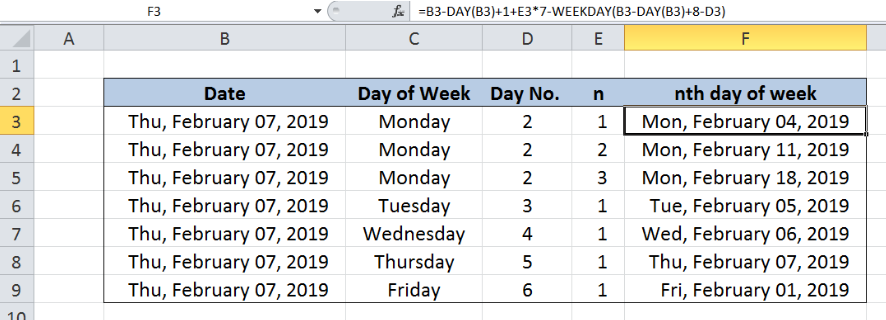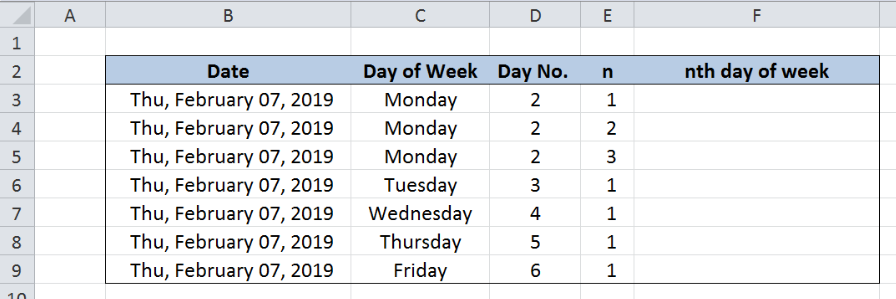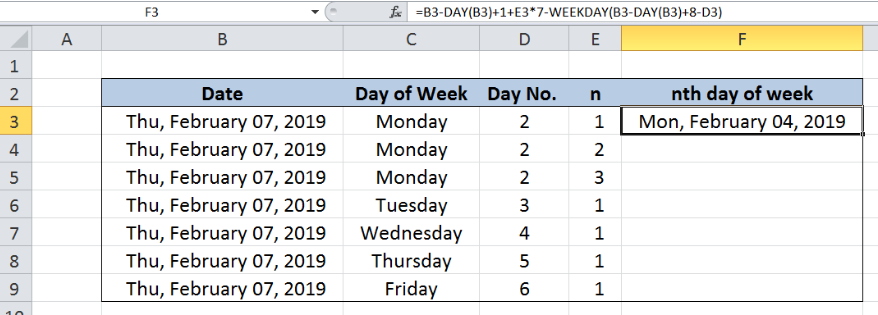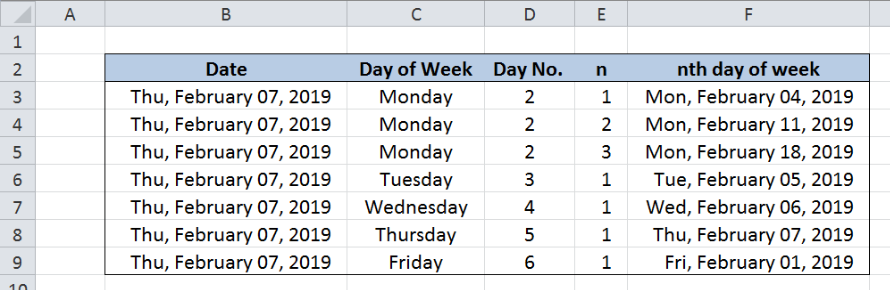Get instant live expert help with Excel or Google Sheets“My Excelchat expert helped me in less than 20 minutes, saving me what would have been 5 hours of work!”

#### Post your problem and you’ll get expert help in seconds.

Your message must be at least 40 characters
Our professional experts are available now. Your privacy is guaranteed.

# How to Get the nth Day of the Week in a Month in Excel

Performing calculations involving dates and day of the week becomes very tedious and often complicated.  However, while working with Excel we are able to find solutions that help us obtain our desired results efficiently.  This step by step tutorial will assist all levels of Excel users in getting the nth day of the week in a month by using the DAY and WEEKDAY functions.Figure 1.  Final result: Get nth day of week in month

Final formula: `=B3-DAY(B3)+1+E3*7-WEEKDAY(B3-DAY(B3)+8-D3)`

## Syntax of DAY Function

DAY function returns an integer corresponding to the date in a month;  values range from 1 to 31

`=DAY(serial_number)`

• Serial_number – a date whose day we want to find; can be a date enclosed in quotation marks or a cell reference
• The date must be between January 1, 1900 and December 31, 9999
• Dates must not be entered as text to avoid errors

## Syntax of WEEKDAY Function

WEEKDAY returns the day of the week of the given date; returns an integer ranging from 1 to 7;

By default, 1 means Sunday and 7 means Saturday

`=WEEKDAY(serial_number,[return_type])`

• serial_number  – the date for which we want to find the corresponding day of the week
• return_type  – Optional; determines the type of return value; if omitted, 1 refers to Sunday and 7 refers to Saturday

## Setting up our Data

Our table consists of five columns: Date (column B), Day of Week (column C), Day No. (column D), n (column E) and nth day of week (column F).  The significance of the date in column B is to determine in which month we want the results to be. The date can actually be any date in the month of choice.  For simplification, we have entered only one date, February 7, 2019.

Our desired day of the week is entered in column C, and the corresponding numbers are entered in column D.  By default, each day of the week is assigned a number by Excel where “1” refers to Sunday and “7” refers to Saturday.

The value for n is entered in column E.  For the first example, we want to determine the 1st Monday of February.  Results will be recorded in column F.Figure 2.  Sample data to get nth day of week in month

## Get 1st Monday of February

In order to get the first Monday of February, we will be using a formula with the DAY and WEEKDAY functions.  Let us follow these steps:

Step 1.  Select cell F3

Step 2.  Enter the formula: `=B3-DAY(B3)+1+E3*7-WEEKDAY(B3-DAY(B3)+8-D3)`

Step 3.  Press ENTER

Step 4.  Copy the formula in F3 to cells F4:F9 by clicking the “+” icon at the bottom-right corner of cell F3 and dragging it downFigure 3.  Entering the formula for 1st Monday of February

Our formula can be separated into three components:

• `B3-DAY(B3)+1 `determines the first day of the month, in this case, February 1, 2019

• `E3*7` added to get n weeks after the 1st day of month

For the first example where n is equal to 1, E3*7 refers to 7 days or one week after the 1st day

• `-WEEKDAY(B3-DAY(B3)+8-D3)` provides the necessary adjustment in order to obtain the desired result; where B3-DAY(B3)+8 refers to the 8th day of the month so February 8, then the day number, D3 is subtracted; WEEKDAY then returns the day of the week of the resulting date

Our formula is cleverly organized such that no matter what day of the week the first day falls in, the result will always obtain the desired day of the week on the nth week of the month.

Below is the breakdown of the formula for our first example:

`B3-DAY(B3)+1 `refers to February 1, 2019

`E3*7` equals 1*7 or 7

`-WEEKDAY(B3-DAY(B3)+8-D3) = -WEEKDAY(February 8 - 2) = WEEKDAY(February 6) = 4`

Our formula becomes `[February 1, 2019] + 7 - 4 = [February 1, 2019] + 3  = [February 4, 2019]`

Finally, the resulting date for the 1st Monday of February is February 4, 2019.

Below table shows the results for the desired nth day of week for the month of February.Figure 4.  Output: Get nth day of week for February

Most of the time, the problem you will need to solve will be more complex than a simple application of a formula or function. If you want to save hours of research and frustration, try our live Excelchat service! Our Excel Experts are available 24/7 to answer any Excel question you may have. We guarantee a connection within 30 seconds and a customized solution within 20 minutes.

### Did this post not answer your question? Get a solution from connecting with the expert.Another blog reader asked this question today on Excelchat:
Solution examplesNeeding help expressing a date based on date range If Date = 01 OCT 2017 - 30 APR 2018 + 380 DAYS If Date = 01 MAY 2018 - 31 JUL 2018 + 270 DAYS If Date = 01 AUG 2018 - 30 SEP 2018 + 430 DAYS
Solved by E. J. in 14 minsneed help with the different sheets and formula IF(A4=B4,0,NETWORKDAYS(A4+1,B4,\$E\$9:\$E\$42) +0.5*(INT((+B4-A4+1)/7)+IF(WEEKDAY(A4+1)+MOD (B4-A4-1,7)>=7,1,0)))
Solved by O. D. in 11 minsNEED A FORMULA TO SHOW THE FOLLOWING: IF (Q3) IS AFTER TODAY & (R3) IS BLANK,THEN "BEHIND SCHEDULE". IF (R3) IS POPULATED THEN "COMPLETE". IF (Q3) IS
Solved by E. U. in 42 minsI need to modify my formula below to automatically the change cell color to yellow if the result in result in cell H3 is greater than 30. This formula is in cell H3 =IF(ISNUMBER(B3), TODAY()-B3, " ")
Solved by O. J. in 39 minsHOW CAN I COUNT NUMBERS PER DAY FOR A MONTH AS TOTAL?
Solved by F. F. in 16 mins## Subscribe to Excelchat.coAnother blog reader asked this question today on Excelchat: